# AP Chemistry : Endothermic and Exothermic Reactions

## Example Questions

### Example Question #1 : Endothermic And Exothermic Reactions

What type of energy conversion occurs during an endothermic reaction?

Nuclear energy to chemical energy

Chemical energy to kinetic energy

Kinetic energy to potential energy

Kinetic energy to chemical energy

Temperature to chemical energy

Kinetic energy to chemical energy

Explanation:

In an endothermic reaction, heat is absorbed and converted into chemical energy. Heat is the sum of molecular kinetic energy in a sample, so kinetic energy is converted into chemical energy. Note that temperature is a measure of heat, and is not a type of energy in itself.

### Example Question #1 : Endothermic And Exothermic Reactions

What will happen to the rate of an exothermic reaction if the temperature is increased?

It will decrease

It will increase

It depends on the initial concentrations of the reactants

It will be unaffected

It will increase

Explanation:

The rate of a reaction will always increase with temperature.

Do not confuse the rate at which a reaction proceeds with the final equilibrium of the reaction. Yes, increasing the temperature of an exothermic reaction will push the equilibrium towards the reactant's side, but this equilibrium will be achieved much more quickly at higher temperatures. Kinetics and thermodynamics are two completely different ways to observe a reaction.

### Example Question #1 : Endothermic And Exothermic Reactions

If a reaction is endothermic and occurs spontaneously, which of the following is not true?

K = 0

ΔH > 0

ΔS > 0

ΔG < 0

K = 0

Explanation:

ΔH is always positive for an endothermic reaction, and ΔG is always negative for a spontaneous reaction. Given the equation delta G = ΔH – T(ΔS), T(ΔS) is positive, so ΔS is positive. We do not know anything about the equilibrium of the reaction.

### Example Question #1 : Endothermic And Exothermic Reactions

If a reaction has a negative entropy and a negative enthalpy value, which of the following terms describes the energy of this reaction?

Exothermic
Exergonic
Endergonic
Constant
Endothermic
Explanation:

Endergonic and exergonic are terms used to describe the free energy of a reaction, so they do not apply in this case since there is not enough information to determine the free energy produced by this reaction without knowing the temperature (delta G = delta H - T delta S). The negative enthalpy allows us to definitively say that the reaction is exothermic.

### Example Question #1 : Endothermic And Exothermic Reactions

Consider the following reversible, exothermic reaction: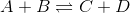The temperature of the vessel in which the reaction is taking place is increased from 300K to 350K. This leads the rate of the reverse reaction to __________.

decrease

stay the same

The change cannot be determined

increase

increase

Explanation:

In exothermic and endothermic reactions, heat can be considered to be either a product or reactant, respectively. Increasing the temperature of the an exothermic reaction is effectively the same as adding heat as a product, causing the reaction to shift to the left. This will cause the reverse reaction to increase in rate.

### Example Question #6 : Endothermic And Exothermic Reactions

If the decomposition of 2 moles of gaseous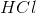into 1 mole each of gaseous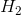and gaseous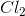at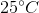absorbs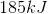of heat energy, then what is the standard heat of formation ofin kilojoules per mole?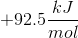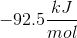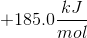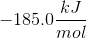Explanation:

From the question stem, we are told that the breakdown of 2 moles of gaseousrequires an absorption ofof energy. For simplification, we can write out the reaction as: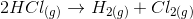By definition, the heat of formation of a compound is the enthalpy change that occurs for the formation (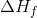) of that compound from its elements in their standard state. Sinceandare in their standard state, we know that they have a heat of formation of zero, thus simplifying the calculation. Thus, if we reverse the above reaction, we see that: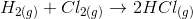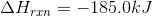Notice that by reversing the reaction, we are also reversing the sign for the enthalpy of the reaction. Now, to calculate theofas kilojoules per mole, we need to multiply the reaction by one-half to obtain: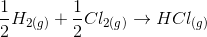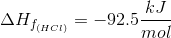### Example Question #1 : Endothermic And Exothermic Reactions

The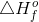of individual products and reactants can be utilized to determine if the reaction is exothermic or endothermic.

Consider the following equation: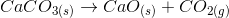Use a standard enthalpy of formation table to calculate the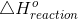, and determine if the reaction is endothermic or exothermic.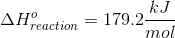, the reaction is exothermic, the reaction is endothermic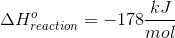, the reaction is exothermic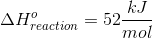, the reaction is exothermic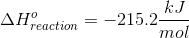, the reaction is endothermic, the reaction is endothermic

Explanation:

We can calculate the standard change in enthalpy for the reaction using the following equation: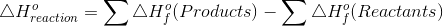of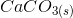of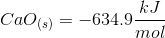of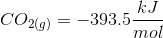Plug in known values and solve.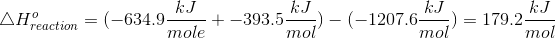A negativeindicates that the reaction is exothermic. This means that the reaction will release energy (usually in the form of heat) to the surroundings.

A positiveindicates that the reaction is endothermic. This means that the reaction will absorb energy (usually in the form of heat) from the surroundings.

Therefore, this overall reaction is endothermic.

### Example Question #8 : Endothermic And Exothermic Reactions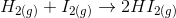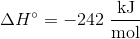The reaction shown is __________, and heat is __________ by the reaction.

exothermic . . . released

endothermic . . . released

endothermic . . . absorbed

exothermic . . . absorbed

Negative enthalpy change(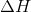) indicates that heat is on the product side of the reaction, or, is released by the reaction. This is also the definition of an exothermic reaction.